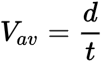## Friday, October 30, 2020

### Uniformly Accelerated Motion

Speed is a scalar quantity. If an object takes a time interval t to travel a distance d, then average speed is equal to the total distance traveled divided by time taken:OrHere the distance is the total (along the path) length traveled. Velocity is a vector quantity. If an object undergoes a vector displacement, s, in a time interval, t, then average velocity is equal to vector displacement divided by timeOrThe direction of the velocity vector is the same as that of the displacement vector. The units of velocity (and speed) are those of distance divided by time, such as m/s or km/h.

Acceleration also a vector quantity, measures the time-rate-of-change of velocity:Where Vi is the initial velocity, Vf, is the final velocity, and t is the time interval over which the change occurred. The units of acceleration are those of velocity divided by time. A typical example is (m/s)/s or ms^2 )